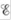# The free energy change for a reaction, Δ G, is an extensive property. What is an extensive property? Surprisingly, one can calculate Δ G from the cell potential. , for the reaction. This is surprising because : is an intensive property. How can the extensive property Δ G be calculated from the intensive property ?### Chemistry: An Atoms First Approach

2nd Edition
Steven S. Zumdahl + 1 other
Publisher: Cengage Learning
ISBN: 9781305079243

#### Solutions

Chapter
Section### Chemistry: An Atoms First Approach

2nd Edition
Steven S. Zumdahl + 1 other
Publisher: Cengage Learning
ISBN: 9781305079243
Chapter 17, Problem 23Q
Textbook Problem
1 views

## The free energy change for a reaction, ΔG, is an extensive property. What is an extensive property? Surprisingly, one can calculate ΔG from the cell potential., for the reaction. This is surprising because: is an intensive property. How can the extensive property ΔG be calculated from the intensive property?

Interpretation Introduction

Interpretation:

The extensive nature of ΔG and its calculation from an intensive property E is given. The definition of extensive property and calculation of extensive property ΔG from an intensive property E is to be stated.

Concept introduction:

The property which depends on the quantity of the substance is called an extensive property, while the quantity which is independent of the quantity of the substance is called an intensive property. The outcome of the multiplication of an extensive property with an intensive property is extensive.

To determine: The definition of an extensive property and the calculation of ΔG from E .

### Explanation of Solution

The property which depends on the quantity of the substance is called an extensive property.

The free energy change for a reaction (ΔG) depends on the quantity of the substance and is therefore an extensive property. It shows the additive nature.

The extensive property ΔG is easily calculated from the formula,

ΔG=-nFEcell

Where,

• ΔG is the Gibbs free energy change

### Still sussing out bartleby?

Check out a sample textbook solution.

See a sample solution

#### The Solution to Your Study Problems

Bartleby provides explanations to thousands of textbook problems written by our experts, many with advanced degrees!

Get Started

Find more solutions based on key concepts
Organic nutrients include all of the following except. a. minerals b. fat c. carbohydrates d. protein

Nutrition: Concepts and Controversies - Standalone book (MindTap Course List)

How many moles are contained in a 54.0g sample of Al? a. 1.0 b. 2.0 c. 0.5 d. 4.0

Chemistry for Today: General, Organic, and Biochemistry

You are standing perfectly still and then take a step forward. Before the step, your momentum was zero, but aft...

Physics for Scientists and Engineers, Technology Update (No access codes included)

What were Matthew Maurys contributions to marine science? Benjamin Franklins?

Oceanography: An Invitation To Marine Science, Loose-leaf Versin